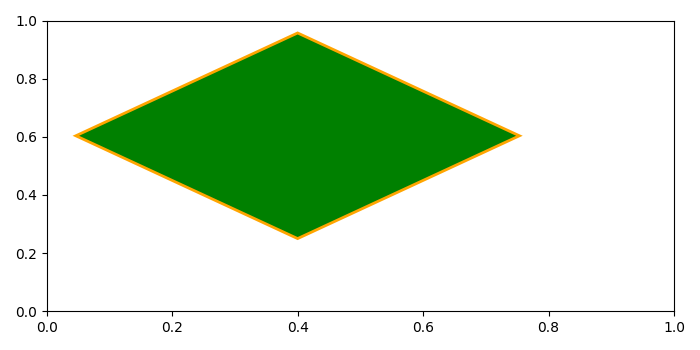# How to rotate the rectangle patch in a plot using Matplotlib?

To rotate the rectangle patch in a plot, we can use angle in the Rectangle() class to rotate it.

## Steps

• Create a figure and a set of subplots using subplots() method.

• Add a rectangle on the patch, angle=45°.

• Add a patch on the axis.

• To display the figure, use show() method.

## Example

from matplotlib import pyplot as plt, patches
plt.rcParams["figure.figsize"] = [7.00, 3.50]
plt.rcParams["figure.autolayout"] = True
figure, ax = plt.subplots(1)
rectangle = patches.Rectangle((0.4, 0.25), 0.5, 0.5, edgecolor='orange',
facecolor="green", linewidth=2, angle=45)
plt.show()